#### 相似文章MetaTrader 4测试者 | 2 二月 2017, 09:267 8400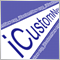### 简介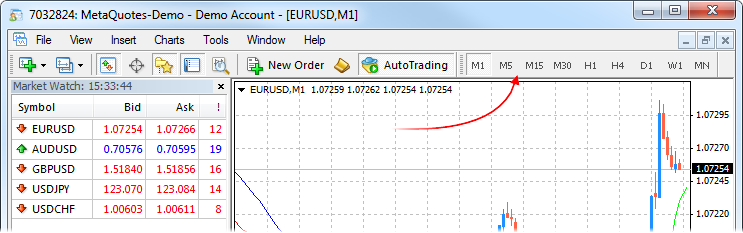### 要点

1. 准备离线工作的额外终端，这样新的标准时间范围数据不会添加到非标准时间范围图表。

2. 利用终端的 period_converter 脚本准备非标准时间范围数据。终端具有足够的可用分钟数据档案。

3. 将准备的数据导入做好测试准备的终端。

1. 准备用于测试非标准时间范围的终端。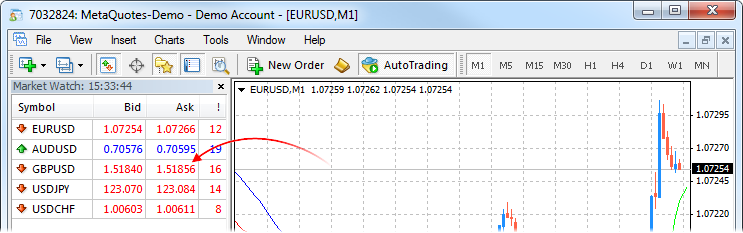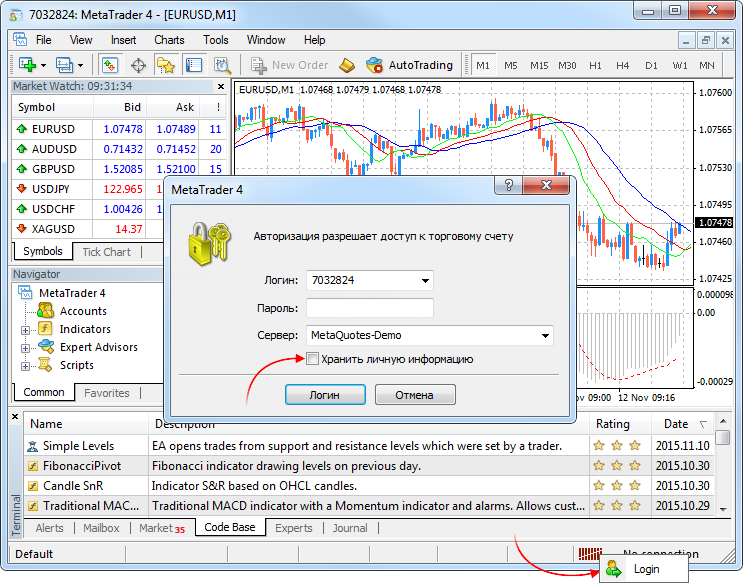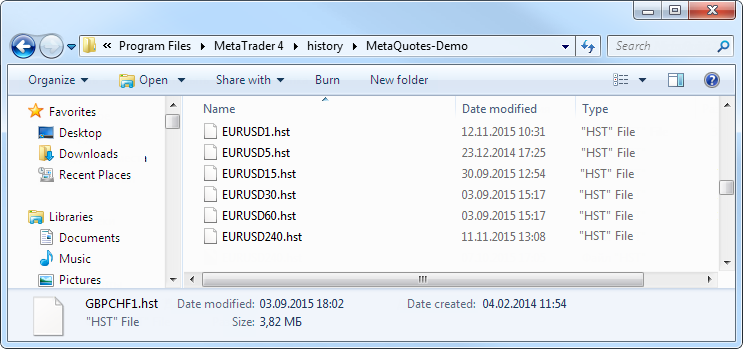2. 准备非标准时间范围数据

"2009.03.19 18:28:09 period_converter EURUSD,M1: 25378 record(s) written"

```int start(){
int h=FileOpen(Symbol()+Period()+".csv",FILE_WRITE|FILE_CSV,",");
for(int i=Bars-1;i>=0;i--){
FileWrite(h,TimeToStr(Time[i],TIME_DATE),TimeToStr(Time[i],TIME_MINUTES),Open[i],High[i],Low[i],Close[i],Volume[i]);
}
FileClose(i);
return(0);
}```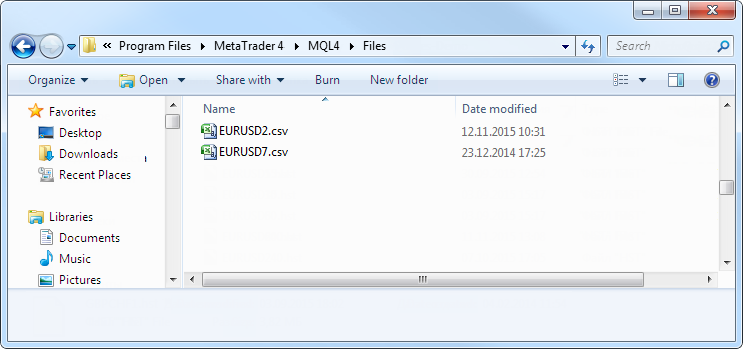3. 导入数据。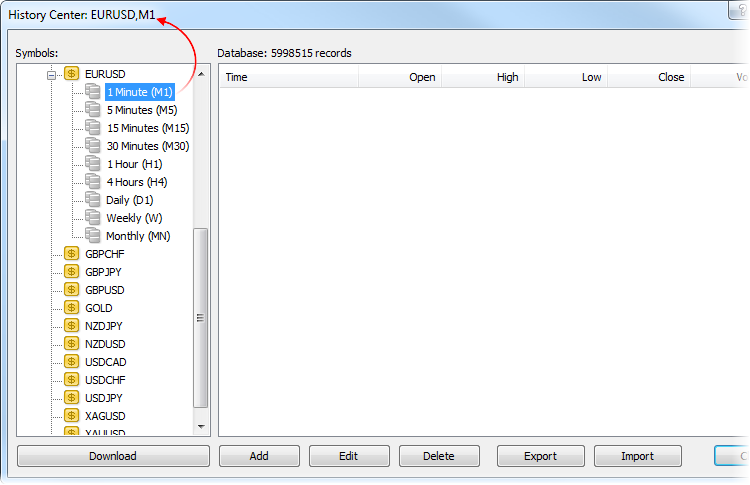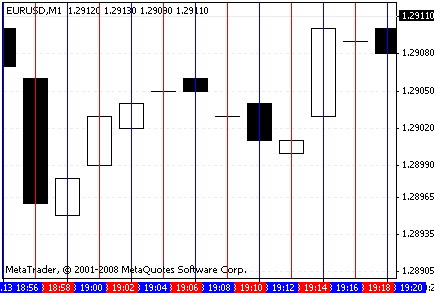EURUSD M5 与之类似——你会看到显示的是 M7 而不是 M5。

4. 检查。

```extern int TimeFrame=0;
extern double Lots=0.1;
extern int FastMAPeriod=13;
extern int FastMAMethod=0;
extern int FastMAPrice=0;
extern int SlowMAPeriod=21;
extern int SlowMAMethod=0;
extern int SlowMAPrice=0;

int start(){
double fast_ma_1=iMA(NULL,TimeFrame,FastMAPeriod,0,FastMAMethod,FastMAPrice,1);
double slow_ma_1=iMA(NULL,TimeFrame,SlowMAPeriod,0,SlowMAMethod,SlowMAPrice,1);
double fast_ma_2=iMA(NULL,TimeFrame,FastMAPeriod,0,FastMAMethod,FastMAPrice,2);
double slow_ma_2=iMA(NULL,TimeFrame,SlowMAPeriod,0,SlowMAMethod,SlowMAPrice,2);
static int bt=0;
static int st=0;
if(fast_ma_1>slow_ma_1){
if(fast_ma_2<=slow_ma_2){
st=0;
}
}
if(fast_ma_1<slow_ma_1){
if(fast_ma_2>=slow_ma_2){
if(bt>0)OrderClose(bt,Lots,Bid,0,CLR_NONE);
bt=0;
if(OrdersTotal()==0)st=OrderSend(Symbol(),OP_SELL,Lots,Bid,0,0,0,"",0,0,CLR_NONE);
}
}
return(0);
}
```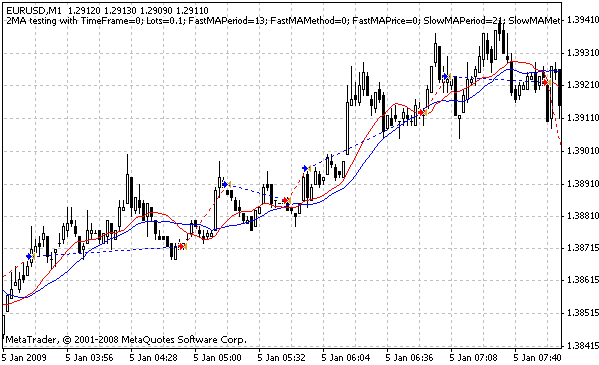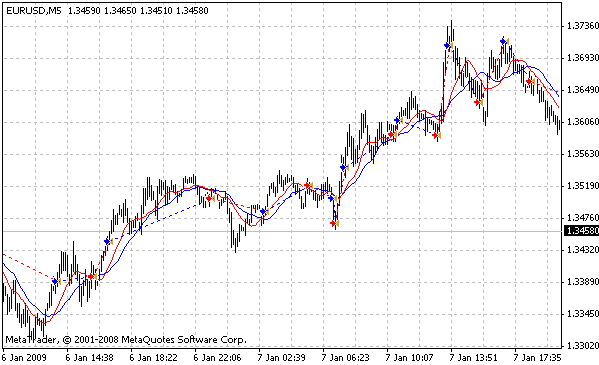```int start(){
double fast_ma_1=iMA(NULL,TimeFrame,FastMAPeriod,0,FastMAMethod,FastMAPrice,1);
double slow_ma_1=iMA(NULL,TimeFrame,SlowMAPeriod,0,SlowMAMethod,SlowMAPrice,1);
double fast_ma_2=iMA(NULL,TimeFrame,FastMAPeriod,0,FastMAMethod,FastMAPrice,2);
double slow_ma_2=iMA(NULL,TimeFrame,SlowMAPeriod,0,SlowMAMethod,SlowMAPrice,2);

double _fast_ma_1=iMA(NULL,MA2TimeFrame,FastMAPeriod,0,FastMAMethod,FastMAPrice,1);
double _slow_ma_1=iMA(NULL,MA2TimeFrame,SlowMAPeriod,0,SlowMAMethod,SlowMAPrice,1);

static int bt=0;
static int st=0;
if(fast_ma_1>slow_ma_1){
if(fast_ma_2<=slow_ma_2){
if(_fast_ma_1>_slow_ma_1){
st=0;
}
}
}
if(fast_ma_1<slow_ma_1){
if(fast_ma_2>=slow_ma_2){
if(_fast_ma_1<_slow_ma_1){
if(bt>0)OrderClose(bt,Lots,Bid,0,CLR_NONE);
bt=0;
if(OrdersTotal()==0)st=OrderSend(Symbol(),OP_SELL,Lots,Bid,0,0,0,"",0,0,CLR_NONE);
}
}
}
return(0);
}
```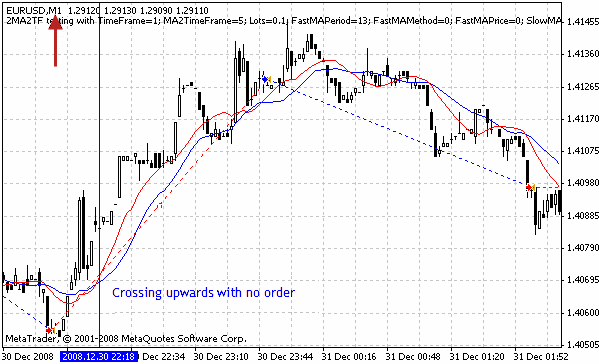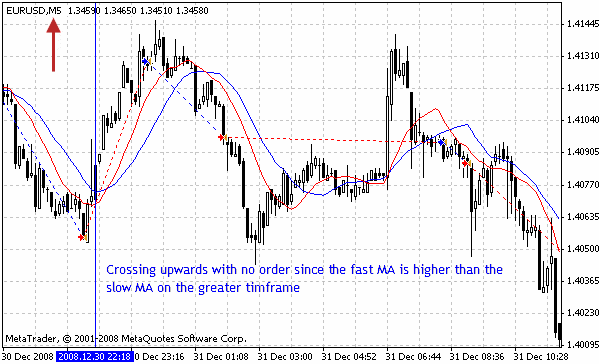### 总结

2MA.mq4 (1.29 KB)
2MA2TF.mq4 (1.64 KB)

#### 该作者的其他文章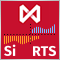一个为莫斯科交易所期货开发的点差策略实例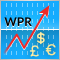交易货币篮子时可用的形态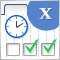图形界面 X: 时间控件, 复选框列表控件和表格排序 (集成编译 6)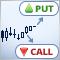如何构建和使用 MetaTrader 4的策略测试器来测试二元期权策略